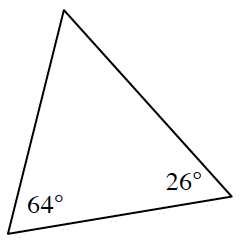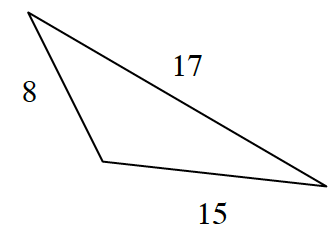### Home > GC > Chapter 10 > Lesson 10.3.1 > Problem10-94

10-94.

For each triangle below, use the information in the diagram to decide if it is a right triangle. Justify each conclusion. Assume the diagrams are not drawn to scale.

1.Use the Triangle Angle Sum Theorem to find the measure of the last angle. Is it a right triangle?

1.• Think of the Pythagorean Theorem. What must be true in order for the triangle to be a right triangle?